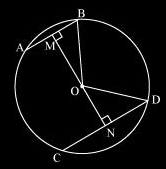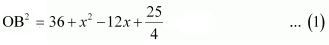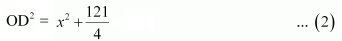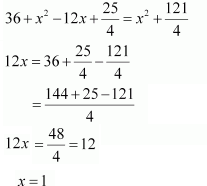Guru

# Two chords AB and CD of lengths 5 cm and 11 cm respectively of a circle are parallel to each other and are on opposite sides of its centre. If the distance between AB and CD is 6 , find the radius of the circle. Q.2

• 0

What is the easiest way for solving the question of class 9th ncert math of exercise 10.6of math of Circles chapter of question no. 2  Please help me for the best suggestion of this question Two chords AB and CD of lengths 5 cm and 11 cm respectively of a circle are parallel to each other and are on opposite sides of its centre. If the distance between AB and CD is 6 , find the radius of the circle.

Share

1.Here, OM ⊥ AB and ON ⊥ CD. is drawn and OB and OD are joined.

We know that AB bisects BM as the perpendicular from the centre bisects chord.

Since AB = 5 so,

BM = AB/2

Similarly, ND = CD/2 = 11/2

Now, let ON be x.

So, OM = 6−x.

Consider ΔMOB,

OB2 = OM2+MB2

Or,Consider ΔNOD,

OD2 = ON2 + ND2

OrWe know, OB = OD (radii)

From equation 1 and equation 2 we getNow, from equation (2) we have,

OD2= 12 +(121/4)

Or OD = (5/2)×√5 cm

• 1